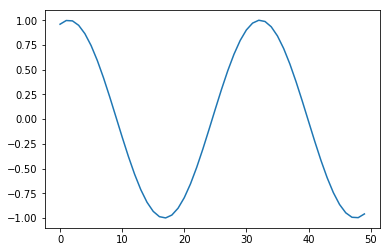# [Notebook] Testing Bokeh Interactive Plot on Nikola Sites

This post shows how to add interactive data visualizations to Nikola-powered websites. Everything will be done in Jupyter notebooks! I use Bokeh as an example but similar approaches should work with other JavaScript-based tools like Plotly. For the impatient, drag to the end of this page to see the results.

For deploying website with Nikola, see my previous post.

In :
!nikola --version

Nikola v8.0.1

In :
%matplotlib inline
import matplotlib.pyplot as plt
import numpy as np


## Some boring stuff to test basic notebook display...¶

Non-executing code snippet:

print('Hello')


Other languages:

if (i=0; i<n; i++) {
printf("hello %d\n", i);
}


Latex: $$e^x=\sum_{i=0}^\infty \frac{1}{i!}x^i$$

Table:

This is
a table
In :
# test matplotlib rendering
plt.plot(np.sin(np.linspace(-5, 5)));Static plots work fine but are a bit boring. Let's create some interactivity.

## Interactive visualization with HvPlot and Bokeh¶

HvPlot provides high-level plotting API built on top of the well-known Bokeh library. Its API is very similar to pandas.DataFrame.plot(), so you don't need to learn too many Bokeh/Holoview-specific syntaxes.

It can be installed easily by pip install hvplot, with all major depedencies.

In :
from IPython.display import HTML

import bokeh
from bokeh.resources import CDN, INLINE
from bokeh.embed import file_html
import holoviews as hv

import pandas as pd
import hvplot.pandas

bokeh.__version__, hv.__version__